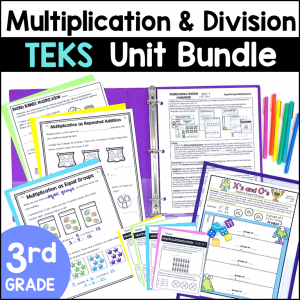Save 10% on your first order with code EXTRA10

# 3rd Grade Multiplication and Division Interactive Notebook – Math TEKS

\$4.00\$29.99
SKU: 8592281 Category:

## Description

This resource includes Easy-to-Use Student Notebook Pages to guide small group and whole class Multiplication and Division lessons. There are engaging pages to teach multiplication and division word problems and concepts. Students learn about arrays, jumps on a number line, the commutative and distributive properties and much more! This notebook becomes a perfect reference tool for students.

This resource has been carefully designed for Texas teachers. In this notebook you will find the TEKS, and nothing but the TEKS. It is designed to be taught cover-to-cover. Your time is precious, and I have done the work of considering the proper weight of topics according to their categorization as readiness or supporting standards. I have also studies the released STAAR tests to understand the format and rigor expected of students.

What’s Included:

• 28 student pages
• Vocabulary section
• Complete Teacher Key

Great ways to use these Notebook Pages:

• Whole Class Lessons
• Small Group Lessons
• Math Stations
• Tutoring

Topics include:

• One-step Multiplication and Division Word Problems
• Arrays
• Representing Multiplication with Repeated Addition, Skip Counting, Jumps on a Number Line, Area Models and Arrays
• Commutative Property of Multiplication
• Distributive Property of Multiplication
• Understanding the Multiplication Chart
• Relationships between Multiplication and Division
• Division of objects into Equal Groups or taking out Equal Sets
• Representing Division with Repeated Subtraction, Skip Counting, Backwards Jumps on a Number Line and Arrays
• Odd and Even Numbers
• Describing Multiplication as a Comparison
• Finding Missing Numbers in Multiplication and Division equations

TEKS Alignment:

Supporting Standards

3.4D: Determine the total number of objects when equally sized groups of objects are combined or arranged in arrays up to 10 by 10.

3.4E: Represent multiplication facts by using a variety of approaches such as repeated addition, equal-sized groups, arrays, area models, equal jumps on a number line, and skip counting.

3.4F: Recall facts to multiply up to 10 by 10 with automaticity and recall the corresponding division facts.

3.4H: Determine the number of objects in each group when a set of objects is partitioned into equal shares or a set of objects is shared equally.

3.4I: Determine if a number is even or odd using divisibility rules.

3.4J: Determine a quotient using the relationship between multiplication and division.

3.5C: Describe a multiplication expression as a comparison such as 3 x 24 represents 3 times as much as 24.

3.5D: Determine the unknown whole number in a multiplication or division equation relating three whole numbers when the unknown is either a missing factor or product.